# ISEE Middle Level Quantitative : Triangles

## Example Questions

← Previous 1 3

### Example Question #1 : Triangles

Which is the greater quantity?

(a) The perimeter of a right triangle with legs of length 5 feet and 12 feet

(b) 8 yards

(b) is greater

(a) and (b) are equal

It is impossible to tell from the information given

(a) is greater

(a) is greater

Explanation:

The length of the hypotenuse of a triangle with legs 5 feet and 12 feet is calculated using the Pythagorean Theorem, setting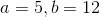: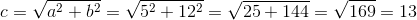The hypotenuse is 13 feet long. The perimeter is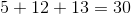feet, which is equal to 10 yards.

### Example Question #2 : Triangles

Which is the greater quantity?

(a) The perimeter of a right triangle with hypotenuse of length 25 centimeters and one leg of length 7 centimeters

(b) One-half of a meter

It is impossible to tell from the information given

(a) and (b) are equal

(a) is greater

(b) is greater

(a) is greater

Explanation:

The length of the second leg of the triangle can be calculated using the Pythagorean Theorem, setting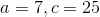: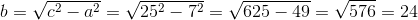The second leg has length 24 centimeters, so the perimeter of the triangle is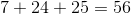centimeters.

One-half of a meter is one-half of 100 centimeters, or 50 centimeters, so (a) is greater.

### Example Question #3 : Triangles

Which is the greater quantity?

(a) The perimeter of an equilateral triangle with sidelength 30 inches

(b) The perimeter of a square with sidelength 2 feet

(a) is greater

(b) is greater

(a) and (b) are equal

It is impossible to tell from the information given

(b) is greater

Explanation:

Each figure has sides that are congruent, so in each case, multiply the sidelength by the number of sides.

(a) The triangle has perimeter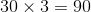inches

(b) 2 feet are equal to 24 inches, so the square has sidelength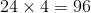inches.

The square has the greater perimeter.

### Example Question #1 : Triangles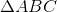is an equilateral triangle;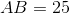.

Rectangle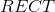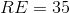Which is the greater quantity?

(a) The perimeter of(b) The perimeter of Rectangle(a) is greater

(b) is greater

It is impossible to tell from the information given

(a) and (b) are equal

It is impossible to tell from the information given

Explanation:

(a) The perimeter of the equilateral triangle is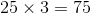.

(b)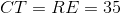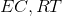are of unknown value, but they are equal,  so we will call their common length.

Rectanglehas perimeter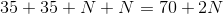.

Without knowing, it cannot be determined with certainty which figure has the longer perimeter. For example:

If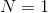, then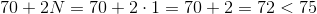If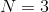, then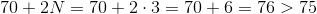### Example Question #1 : Trianglesis an isosceles triangle;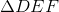is an equilateral triangle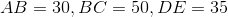Which is the greater quantity?

(a) The perimeter of(b) The perimeter of(b) is greater

It is impossible to tell from the information given

(a) and (b) are equal

(a) is greater

(a) is greater

Explanation:

(a) As an isosceles triangle,, by definition, has two congruent sides.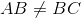, so either :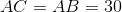in which case the perimeter ofis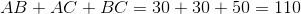or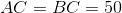in which case the perimeter ofis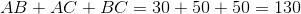(b)is an equilateral triangle, so, by definition, all of its sides are congruent; its perimeter is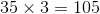.

Regardless of the length of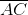has the greater perimeter.

### Example Question #6 : Trianglesandare right triangles, with right angles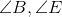, respectively.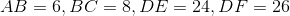Which is the greater quantity?

(a)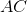(b)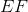(b) is greater

(a) is greater

It is impossible to tell from the information given

(a) and (b) are equal

(a) and (b) are equal

Explanation:

(a)is the hypotenuse of, so by the Pythagorean Theorem,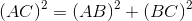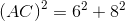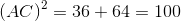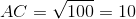(b)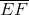is a leg of, whose hypotenuse is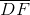, so by the Pythagorean Theorem,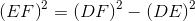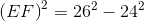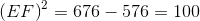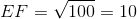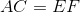### Example Question #631 : Isee Middle Level (Grades 7 8) Quantitative Reasoning

Which is the greater quantity?

(a) The perimeter of a right triangle with legs of length 3 inches and 4 inches

(b) One foot

It is impossible to tell from the information given

(a) and (b) are equal

(b) is greater

(a) is greater

(a) and (b) are equal

Explanation:

The length of the hypotenuse of a triangle with legs 3 inches and 4 inches long is calculated using the Pythagorean Theorem, setting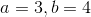: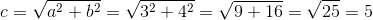The hypotenuse is 5 inches long. The perimeter is therefore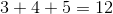inches, which is equal to one foot.

### Example Question #2 : Trianglesis a right triangle with hypotenuse10 inches long.

The lengths of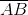and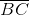, in inches, can both be expressed as integers.

Which is the greater quantity?

(a)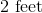(b) The perimeter ofIt is impossible to tell from the information given

(b) is greater

(a) and (b) are equal

(a) is greater

(a) and (b) are equal

Explanation:

By the Pythagorean Theorem,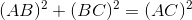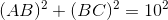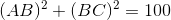By trial and error, it can be determined that the only two positive integers that can replace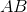and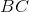to make this equation true are 6 and 8, in either order: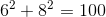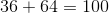Add the three side lengths to get the perimeter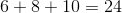inches, which is equal to 2 feet.

### Example Question #9 : Triangles

An equilateral triangle has perimeter two yards. Which is the greater quantity?

(A) The length of one side of the triangle

(B) 28 inches

(B) is greater

It is impossible to determine which is greater from the information given

(A) is greater

(A) and (B) are equal

(B) is greater

Explanation:

An equilateral triangle has three sides of equal length; the perimeter of this triangle is two yards, which is equal to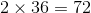inches. One side has length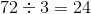inches, which is less than 28 inches, so (B) is greater.

### Example Question #10 : Triangles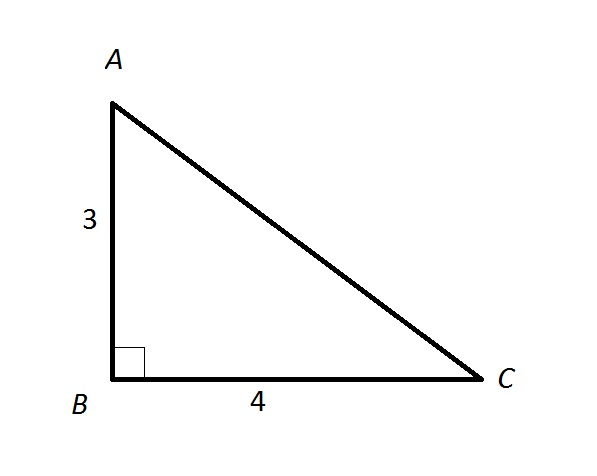Note: Figure NOT drawn to scale.

Refer to the above triangle. An insect walks directly from B to A, then directly from A to C. What percent of the perimeter of the triangle has he walked?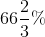It is impossible to answer the question from the information given.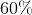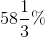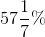Explanation:

By the Pythagorean Theorem, the distance from A to C, which we will call, is equal to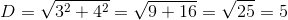The perimeter of the triangle is. The insect has traveled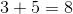units out of 12, which is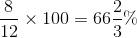of the perimeter.

← Previous 1 3